# FKG inequality

Jump to: navigation, search

Fortuin–Kasteleyn–Ginibre inequality

An inequality [a3] that began a series of correlation inequalities for finite partially ordered sets. Letbe a finite partially ordered set ordered by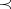(irreflexive, transitive) witha distributive lattice:,, and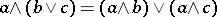for all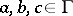. Suppose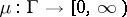is log supermodular:and thatandare non-decreasing:The FKG inequality is: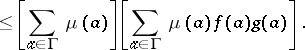Ifis a Boolean algebra andis a probability measure on, the inequality is, where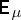denotes mathematical expectation.

Related inequalities are discussed in [a1], [a2], [a4], [a5], [a6], [a7], [a8], [a9].